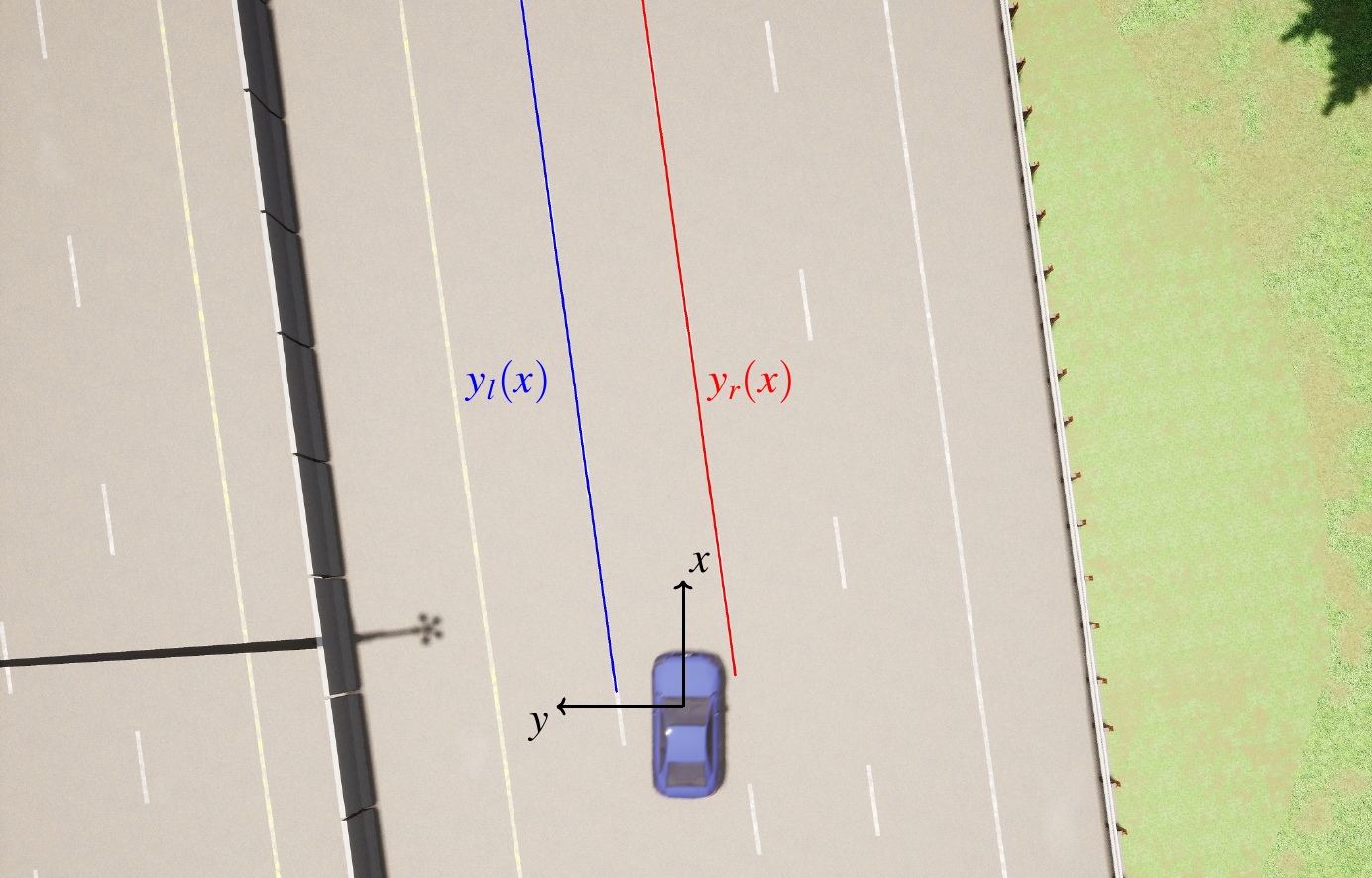# Overview¶

## Lane Detection System¶

In this chapter, I will guide you to build a simple but effective lane detection pipeline and apply it to images captured in the Carla Simulator. The pipeline takes an image as input and yields a mathematical model of the lane boundaries as an output. The image is captured by a dashcam - a camera that is fixated behind the windshield of a vehicle. The lane boundary model is a polynomial

$y(x)=c_0+c_1 x+c_2 x^2 +c_3 x^3$

Here, both $$x$$ and $$y$$ are measured in meters. They define a coordinate system on the road as shown in Fig. 1.Fig. 1 Road coordinate system. The perspective is known as bird’s eye view.

The pipeline consists of two steps

• Using a neural network, detect those pixels in an image that are lane boundaries

• Associate the lane boundary pixels to points on the road, $$(x_i,y_i), i=0,1,2\dots$$. Then fit a polynomial.

The approach is inspired by the “baseline” method described in Ref. [GBN+19], which performs close to state-of-the-art lane-detection methods.

## Lane Boundary Segmentation - Deep Learning¶

In the chapter Lane Boundary Segmentation, we will train a neural network, which takes an image and estimates for each pixel the probability that it belongs to the left lane boundary, the probability that it belongs to the right lane boundary, and the probability that it belongs to neither. As you might know, a neural network needs data to learn. Luckily, it is easy to gather this data using the Carla simulator: We are going to create a vehicle on the Carla map and attach an rgb camera sensor to it. Then we will move the vehicle to different positions on the map and capture images with our camera. The 3d world coordinates of the lane boundaries are obtained from the Carla simulator’s high definition map and can be projected into the image using the pinhole camera model.Fig. 2 The Lane boundaries from Carla’s HD map are projected into the dashcam image (blue and orange).

For each simulation step, we save two separate images:

• The image captured by the dashcam

• A label image that only consists of the projected lane boundaries

You will learn how to create the label images in the chapter Basics of image formation.

## From pixels to meters - Inverse Perspective Mapping¶

A camera maps the three-dimensional world into a two-dimensional image plane. In general, it is not possible to take a single image and to reconstruct the three-dimensional coordinates of the objects depicted in that image. Using the pinhole camera model, we can reconstruct from which direction the light ray came, that was scattered off the depicted object, but not how many meters it has traveled. This is different for the light that was scattered from the road into our camera sensor. Using the assumption that the road is flat, and our knowledge of the camera height and orientation with respect to the road, it is a basic geometry problem to compute the $$x,y,z$$ position of each “road pixel” ($$x,y,z$$ in meters!). This computation is known as inverse perspective mapping and you will learn about it in the chapter From Pixels to Meters.

From our deep learning model we have a list of probabilities $$p_i(\textrm{left boundary}), i=0,1,2, \dots$$ for all the pixels. Using inverse perspective mapping we can even write down a list of tuples $$(x_i,y_i,p_i(\textrm{left boundary})), i=0,1,2, \dots$$, since we know the road coordinates $$(x_i,y_i)$$ of each pixel.

We can now filter this list and throw away all tuples where $$p_i(\textrm{left boundary})$$ is small. The filtered list of $$(x_i,y_i)$$ can be fed into a method for polynomial fitting, which will result in a polynomial $$y(x)=c_0+c_1 x+c_2 x^2 +c_3 x^3$$ describing the left lane boundary. The same procedure is repeated for the right boundary.

## Outlook¶

Once you have finished all exercises of this chapter, you will have implemented a python class LaneDetector that can yield lane boundary polynomials $$y_l(x), y_r(x)$$ for the left and right lane boundary, given an image from a dashcam in the Carla simulator. In the next chapter, we will write a lane-keeping system for a vehicle in the Carla simulator. This lane-keeping system needs the desired speed and a reference path as inputs. You can take the centerline between the lane boundaries that your LaneDetector class computes, and feed that into your lane-keeping system.